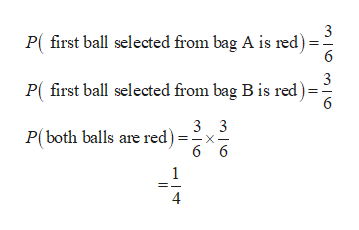Assume that each bag contains 6 balls. Bag a contains 3 red and 3 white, while bag b contains 2 red, 2 white, and 2 blue. You randomly select one ball from bag a, note the color, and place the ball in bag b. You then select a ball from bag b at random and make note of its color.(1) What is the probability that both balls are red? (2) What is the probability that both balls are red given that the first ball you drew was red?

Question

Assume that each bag contains 6 balls. Bag a contains 3 red and 3 white, while bag b contains 2 red, 2 white, and 2 blue. You randomly select one ball from bag a, note the color, and place the ball in bag b. You then select a ball from bag b at random and make note of its color.

(1) What is the probability that both balls are red?

(2) What is the probability that both balls are red given that the first ball you drew was red?

Step 1

Given that each bag contains 6 balls, Bag A contains 3 red and 3 white while Bag B contains 2 red and 2 white and 2 blue.

Step 2

1) Obtain the probability that both the balls are red as,help_outlineImage Transcriptionclose3 P(first ball selected from bag A is red) 6 3 P( first ball selected from bag B is red) 6 3 3 P(both balls are red) 6 6 4 X fullscreen
Step 3

2) Obtain the probability that both the balls a...

Want to see the full answer?

See Solution

Want to see this answer and more?

Our solutions are written by experts, many with advanced degrees, and available 24/7

See Solution
Tagged in

Math